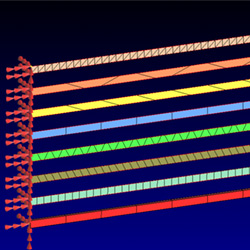WRITTEN BY:

We have all learnt through our careers with FEA (Finite Element Analysis) that "tria3 elements are bad" and that "quad4 elements should stay square" for better results but how much worse are tria3 elements (and under which circumstances?) and exactly how bad is a deformed quad4 element when it comes to the results we obtain from them?

During a NAS120 course I thought it best to demonstrate this to the class.

Let's just look at this simple comparison where I took a number of identical beams and evaluated their stiffness by only changing the element type and shape to represent them.

The beams I chose was 500mm long, 10mm in height and 5mm wide and made of normal mild steel.

As a baseline, I chose beam elements (beam 1) and used Nastran's CBEAM element formulation with the coupled mass method to reduce approximation inaccuracies.

I could have used a tip force (F) and measure the tip displacement (x) to quantify the "bending" stiffness (k) where F=kx but chose not to, or let me rather say I was forced to use the normal modes method to evaluate the beam stiffness. I say forced because the element quality I forced on the meshes were so bad that MSC Nastran would not allow a static analysis while a normal modes analysis was still possible.

Since wn = sqrt(k/m) and the mass (m) was constant for all the beams, the natural frequency (wn) would also be a direct indicator of each beam's stiffness (k).

In this model all the beams were evaluated simultaneously and they showed a consistent first mode of bending around the thinnest dimension (width) as displayed below left and a second mode of bending about the vertical (height) dimension on the right.

When comparing the calculated natural frequencies below, it shows a clear indication of which beams have the same stiffness as beam 1 (in my opinion the most accurate answer) and which ones produced an answer the furthers from it (beam 7).

To put these differences in perspective, a percentage error was calculated here with beam 1 as the reference:

1. This table clearly shows us what we already knew about the Tri elements (beam 8), i.e. that they are quite stiff in shear (modes 2 and 4) but not that stiff in bending (modes 1 and 3) compared to the quad4's (beam 2).
2. What came as a surprise to me was that the highly distorted quad4 elements (beam 7) gave worse answers than the tri elements.
3. What was also quite interesting was that beam 6, which is also made up of highly distorted quad4 elements, did not nearly perform nearly as bad as beam 7.
4. Beam 3 which has skew quad4 elements with a good aspect ratio still gave good results but beam 4 with the same amount of skewness, but in taper form, performed quit badly in shear.

These results made me appreciate the definition of FEA again: "FEA is an approximation method". We can thus not expect absolute answers from FEA but blindly using any element or shape of element can drastically degrade the usefulness of the answers. It is therefore a very good practice to know the element you use by checking its behaviour under different circumstances.